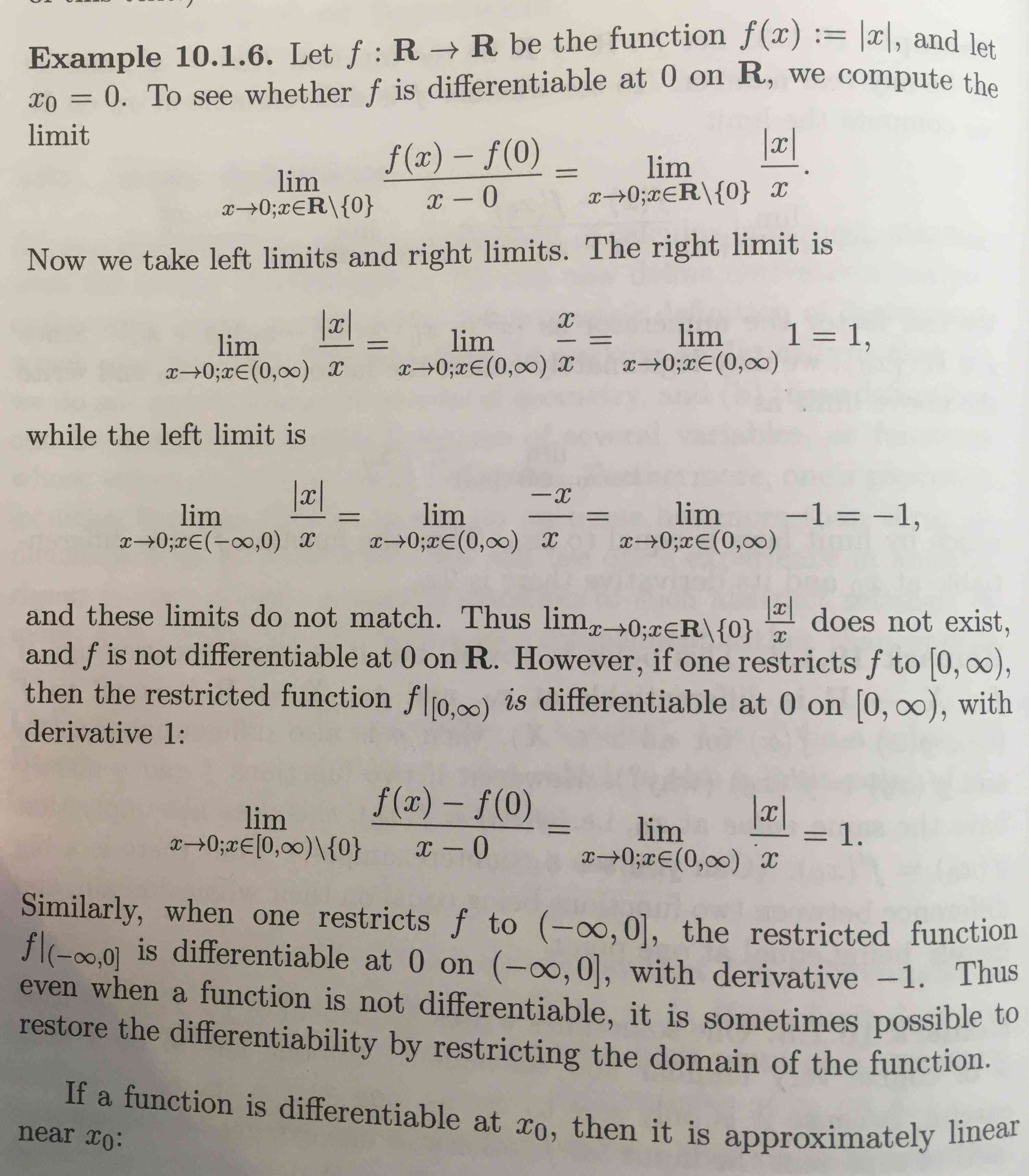Show Question
Math and science::Analysis::Tao::10: Differentiation of functions

# Differentiability at a point, definition

### Differentiability at a point

Let $$X$$ be a subset of $$\mathbb{R}$$, and let $$x_0 \in X$$ be an element of $$X$$ that is also a limit point of $$X$$. Let $$f : X \to \mathbb{R}$$ be a function. Then, if the following limit:

$\lim_{x \to x_0; x \in X \setminus \{x_0\} } \frac{f(x) - f(x_0)}{x - x_0}$

converges to some $$L$$ then we say that $$f$$ is differentiable at $$x_0$$ on $$X$$ with derivative $$L$$. We write: $$f'(x_0) := L$$.

If $$x_0$$ is not an element of $$X$$ or is not a limit point of $$X$$, or the limit does not converge, we leave $$f'(x_0)$$ undefined and we say that $$f$$ is not differentiable at $$x_0$$ on $$X$$.

We need $$x_0$$ to be a limit point (not isolated) in order for it to be adherent to $$X \setminus \{x_0\}$$ and in turn for the above limit to be defined. Thus, functions do not have a derivative defined at isolated points.

### Reasons against the notation $$\frac{df}{dx}$$

Consider two functions $$f : X \to \mathbb{R}$$, defined by $$f(x) := x^2$$ and $$g : X \to \mathbb{R}$$, defined by $$g(y) := y^2$$. We have $$f = g$$. If we write $$\frac{df}{dx}$$ instead of $$f'$$, then it is troublesome to consider $$\frac{dg}{dx}$$, is it 0? Introducing the notion of differentiation along vector fields can resolve this ambiguity, but this is out of scope here.

### Example

Let $$f : \mathbb{R} \to \mathbb{R}$$ be the function $$f(x) := x^2$$, and let $$x_0$$ be any real number. To see whether $$f$$ is differentiable at $$x_0$$ on $$\mathbb{R}$$, we compute the limit:

\begin{aligned} \lim_{x \to x_0; x \in X \setminus \{x_0\} } \frac{f(x) - f(x_0)}{x - x_0} &= \lim_{x \to x_0; x \in X \setminus \{x_0\} } \frac{x^2 - x_0^2}{x - x_0} \\ &= \lim_{x \to x_0; x \in X \setminus \{x_0\} } \frac{(x + x_0)(x - x_0)}{x - x_0} \\ &= \lim_{x \to x_0; x \in X \setminus \{x_0\} } (x + x_0) \\ &= 2 x_0 \\ \end{aligned}

Thus $$f$$ is differentiable at $$x_0$$ on $$\mathbb{R}$$ and its derivative is $$2 x_0$$.

Another example:p252